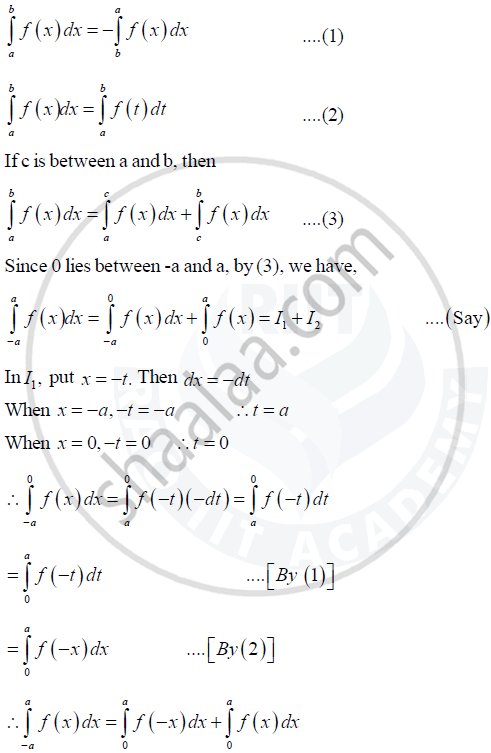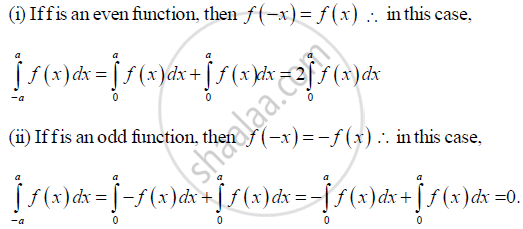Share

# Prove that : ∫a−a f(x)dx=2∫a0f(x)dx , = 0, if f (x) is an odd function. - Mathematics and Statistics

#### Questions

Prove that : int_-a^af(x)dx=2int_0^af(x)dx , if f (x) is an even function.

= 0,                   if f (x) is an odd function.

Show that: int_-a^af(x)dx=2int_0^af(x)dx , if f (x) is an even function.

= 0,                   if f (x) is an odd function.

#### Solution 1

int_-a^af(x)dx=int_-a^0f(x)dx+int_0^af(x)dx

int_a^af(x)dx=I+int_0^af(x)dx

Now I=int_-a^0f(x)dx

Put x=-t

dx = - dt
When x = -a, t = a and when x = 0, t = 0

I=int_a^0f(-t)(-dt)

=-int_a^0f(-t)dt

=int_0^af(-t)dt ...........[because int_a^bf(x)dx=-int_b^af(x)dx]

=int_0^af(-x)dt ...........[because int_a^bf(x)dx=-int_b^af(t)dx]

Equation (i) becomes

int_-a^af(x)dx=int_0^af(-x)dx+int_0^af(x)dx

=int_0^a[f(-x)+f(x)]dx.......(ii)

case 1: If f(x) is an even function, then f(-x) = f(x).
Thus, equation (ii) becomes

int_-a^af(x)dx=int_0^a[f(x)+f(x)]dx=2int_0^af(x)dx

Case 2: If f(x) is an odd function, then f(-x) = -f(x).
Thus, equation (ii) becomes

int_-a^af(x)dx=int_0^a[-f(x)+f(x)]dx=0

#### Solution 2

We shall use the following results :Is there an error in this question or solution?

#### APPEARS IN

2014-2015 (October) (with solutions)
Question 5.2.3 | 4.00 marks
2016-2017 (July) (with solutions)
Question 5.2.3 | 4.00 marks
2017-2018 (March) (with solutions)
Question 5.2.3 | 4.00 marks

#### Video TutorialsVIEW ALL 

Solution Prove that : ∫a−a f(x)dx=2∫a0f(x)dx , = 0, if f (x) is an odd function. Concept: Methods of Integration - Integration by Parts.
S